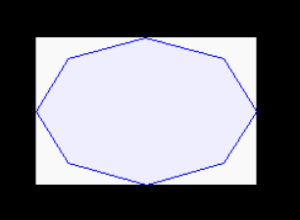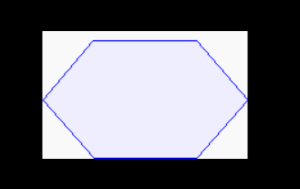# Python PIL | ImageDraw.Draw.polygon() Method

• Last Updated : 27 Aug, 2019

PIL is the Python Imaging Library which provides the python interpreter with image editing capabilities. The `ImageDraw` module provide simple 2D graphics for Image objects. You can use this module to create new images, annotate or retouch existing images, and to generate graphics on the fly for web use.

Attention geek! Strengthen your foundations with the Python Programming Foundation Course and learn the basics.

To begin with, your interview preparations Enhance your Data Structures concepts with the Python DS Course. And to begin with your Machine Learning Journey, join the Machine Learning - Basic Level Course

`ImageDraw.Draw.polygon()`Draws a polygon.
The polygon outline consists of straight lines between the given coordinates, plus a straight line between the last and the first coordinate.

Syntax: PIL.ImageDraw.Draw.polygon(xy, fill=None, outline=None)

Parameters:

Parameters:

xy – Sequence of either 2-tuples like [(x, y), (x, y), …] or numeric values like [x, y, x, y, …].

outline – Color to use for the outline.

fill – Color to use for the fill.

Returns: An Image object.

 `  ` ` ` `import` `math``from` `PIL ``import` `Image, ImageDraw``from` `PIL ``import` `ImagePath `` ` `side ``=` `8``xy ``=` `[``    ``((math.cos(th) ``+` `1``) ``*` `90``,``     ``(math.sin(th) ``+` `1``) ``*` `60``)``    ``for` `th ``in` `[i ``*` `(``2` `*` `math.pi) ``/` `side ``for` `i ``in` `range``(side)]``    ``]  `` ` `image ``=` `ImagePath.Path(xy).getbbox()  ``size ``=` `list``(``map``(``int``, ``map``(math.ceil, image[``2``:])))`` ` `img ``=` `Image.new(``"RGB"``, size, ``"# f9f9f9"``) ``img1 ``=` `ImageDraw.Draw(img)  ``img1.polygon(xy, fill ``=``"# eeeeff"``, outline ``=``"blue"``) `` ` `img.show()`

Output:Another Example:taking different parameters.

 `  ` ` ` `import` `math``from` `PIL ``import` `Image, ImageDraw``from` `PIL ``import` `ImagePath `` ` `side ``=` `6``xy ``=` `[``    ``((math.cos(th) ``+` `1``) ``*` `90``,``     ``(math.sin(th) ``+` `1``) ``*` `60``)``    ``for` `th ``in` `[i ``*` `(``2` `*` `math.pi) ``/` `side ``for` `i ``in` `range``(side)]``    ``]  `` ` `image ``=` `ImagePath.Path(xy).getbbox()  ``size ``=` `list``(``map``(``int``, ``map``(math.ceil, image[``2``:])))`` ` `img ``=` `Image.new(``"RGB"``, size, ``"# f9f9f9"``) ``img1 ``=` `ImageDraw.Draw(img)  ``img1.polygon(xy, fill ``=``"# eeeeff"``, outline ``=``"blue"``) `` ` `img.show()`

Output:My Personal Notes arrow_drop_up